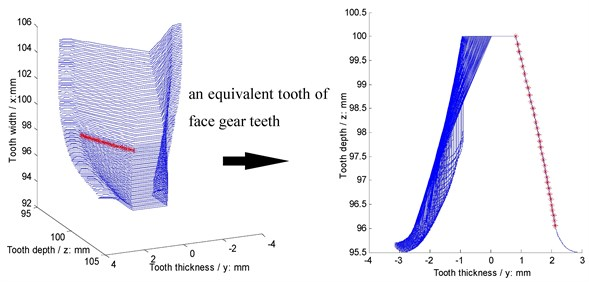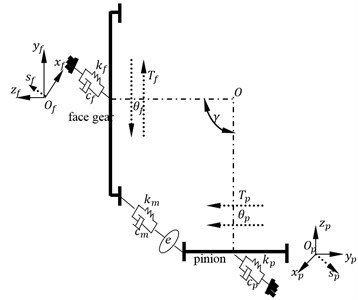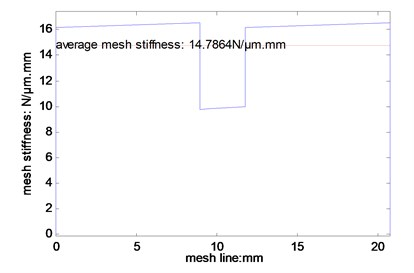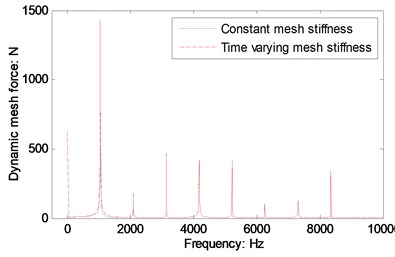Published: 18 September 2015

# Differences of dynamic behaviors of face gear drives between time varying and average mesh stiffness

Zhengminqing Li1
Bei Zhou2
Xiaodong Xu3
Rupeng Zhu4
1, 3, 4Nanjing University of Aeronautics and Astronautics, Nanjing, China
2China Academy of Launch Vehicle Technology, Beijing, China
Corresponding Author:
Zhengminqing Li
Views 20

#### Abstract

Face gear dynamics is one of important studies of face gear drives. However, differences of dynamic behaviors of face gear drives between time varying and average mesh stiffness are not to be discussed. Thus, in this study, a mesh stiffness calculation solution of face gear drives is constructed, which is based on the proposed equivalent face gear teeth, and a four DOF dynamic model of face gear drives is formulated. Furthermore, differences of dynamic behaviors of face gear drives between time varying and average mesh stiffness are investigated. The result indicates instantaneous impacts are existed at start engagement points of face gear drives.

## 1. Introduction

Face gear drives, due to insensitive manufacture and alignment errors versus spiral bevel gear drives [1-5], are addressed by many scholars, and some research achievements of face gear dynamics are obtained by scholars [6-11]. However, according to the limited published issues, differences between the impact of time varying mesh stiffness on dynamic mesh forces of face gear drives and that of average mesh stiffness on dynamic mesh forces are not to be discussed. Thus, in this study, a mesh stiffness calculation solution of face gear drives, based on the proposed equivalent face gear teeth, is constructed, and a four DOF dynamic model of face gear drives is established. The differences of dynamic behaviors of face gear drives between time varying and average mesh stiffness are investigated. The result indicates an instantaneous impact is existed at start engagement points of face gear drives.

## 2. Constructed mesh stiffness calculation solution and four DOF dynamic model

### 2.1. Constructed mesh stiffness calculation solution

A face gear tooth is a kind of gear tooth associated with variable tooth thicknesses, and tooth pointing is produced at extreme outer radii of face gears, and undercut is observed at extreme inner radii of face gears. Thus, a face gear tooth can be assumed as a sequence in which modification involute gears are superimposed along its face width according to geometric characteristics of face gear teeth. Meanwhile, In order to avoid offset load phenomenon of face gear drives, tooth numbers of a pinion, which meshes with a face gear, are always less one to three teeth than a generation gear, which is employed to generate face gears. Therefore, according to the above assumption proposed and the working characteristics, face gear drives can be equivalent to involute modification gear drives based on mesh viewpoints, and an evolutionary procession of equivalent face gear teeth can be expressed as shown in Fig. 1.

As given in Fig. 1, according to the proposed equivalent face gear drives, a mesh stiffness calculation solution of face gear drives can be constructed by traditional Ishikawa model [12, 13].

### 2.2. Four DOF dynamic model

A four DOF dynamic model of face gear drives, as given in Fig. 2, is established for evaluating differences of face gear dynamic behaviors between time varying and average mesh stiffness.

Fig. 1An evolution diagram of equivalent face gear teethFig. 2A four-DOF dynamic model of face gear drivesAs shown in Fig. 2, mathematic equations of the dynamic model can be derived by:

1
$\left\{\begin{array}{l}{m}_{p}{s}_{p}^{\text{'}\text{'}}+{c}_{p}{s}_{p}^{\text{'}}+{k}_{p}{s}_{p}=-{F}_{m},\\ {m}_{f}{s}_{f}^{\text{'}\text{'}}+{c}_{f}{s}_{f}^{\text{'}}+{k}_{f}{s}_{f}={F}_{m},\\ {I}_{p}{\theta }_{p}^{\text{'}\text{'}}+{F}_{m}{R}_{bp}={T}_{p},\\ {I}_{f}{\theta }_{f}^{\text{'}\text{'}}+{F}_{m}{R}_{bf}=-{T}_{f},\end{array}\right\$

where ${F}_{m}$ can be expressed as:

2
${F}_{m}={k}_{m}sin\left(\gamma \right)\left({s}_{p}-{s}_{f}+{R}_{bp}{\theta }_{p}-{R}_{bf}{\theta }_{f}-e\right)$
$+{c}_{m}sin\left(\gamma \right)\left({s}_{p}^{\text{'}}-{s}_{f}^{\text{'}}+{R}_{bp}{\theta }_{p}^{\text{'}}-{R}_{bf}{\theta }_{f}^{\text{'}}-e\text{'}\right),$

where $\theta$ is a torsion degree of freedom, $s$ is a bending degree of freedom, $T$ is a torsion, $k$ is a bending stiffness, $c$ is a bending damping, $m$ is a quality, $I$ is a moment of inertia, ($\text{'}$) is first derivative, ($\text{'}\text{'}$) is second derivative, subscript $f$ and $p$ express a face gear and a pinion respectively. In addition, ${k}_{m}$ is mesh stiffness, ${c}_{m}$ is mesh damping, and $e$ is a comprehensive meshing error.

## 3. Simulations

In order to assess differences between the impact of time varying mesh stiffness on dynamic mesh forces and that of average mesh stiffness on dynamic mesh forces, geometric parameters, operating conditions and material characteristics of an example case of face gear drives are given in Table 1.

Table 1Parameters of an example case

 Names Values Units Geometric parameters Modulus 4 mm Pressure angle 20 ° Tooth number of pinions 21 – Drive ratio 2 – Shaft angle 90 ° Tooth number difference between pinions and generation gears 1 – Addendum coefficient 1 – Clearance coefficient 0.25 – Operating conditions Power 50 kW Input rotation speed 3000 r/min Material characteristics Modulus of elasticity 210000 MPa Poisson ratio 0.3 –

According to the proposed mesh stiffness calculation solution, and the parameters listed in Table 1, the time varying mesh stiffness and average mesh stiffness of the example case are simulated, as shown in Fig. 3. Moreover, the differences of dynamic mesh forces of face gear drives of the example case between time varying and average mesh stiffness, as shown in Fig. 4, are discussed.

Fig. 3Mesh stiffness simulatedFig. 4A diagram of the simulated differencesIn the case of Fig. 4, a zero frequency incentive of dynamic mesh forces, which is the most important difference of dynamic behaviors between time varying and average mesh stiffness, is obtained. The result indicates a transitory shock at start engagement points of face gear drives is existed. Except the above phenomenon, the other differences almost can be neglected according to the simulation.

## 4. Conclusions

In this study, two important works can be extracted as follows:

1) A mesh stiffness calculation solution of face gear drives is constructed, which is based on the proposed equivalent face gear teeth.

2) Differences of dynamic behaviors of face gear drives between time varying and average mesh stiffness are obtained, and the result indicates an instantaneous impact is existed at start engagement points of face gear drives.

These contributions would be helpful to improve engineering applications of face gear drives.

Accepted
04 June 2015
Published
18 September 2015
SUBJECTS
Mechanical vibrations and applications
Keywords
equivalent teeth
face gear drives
time varying mesh stiffness
dynamic mesh force
Acknowledgements

The authors are grateful for the financial support provided by the National Natural Science Foundation of China under No. 51105194 and No. 51375226, and the Fundamental Research Funds for the Central Universities under No. NS2015049.

Conflict of interest

The authors declare that there is no conflict of interests regarding the publication of this article.